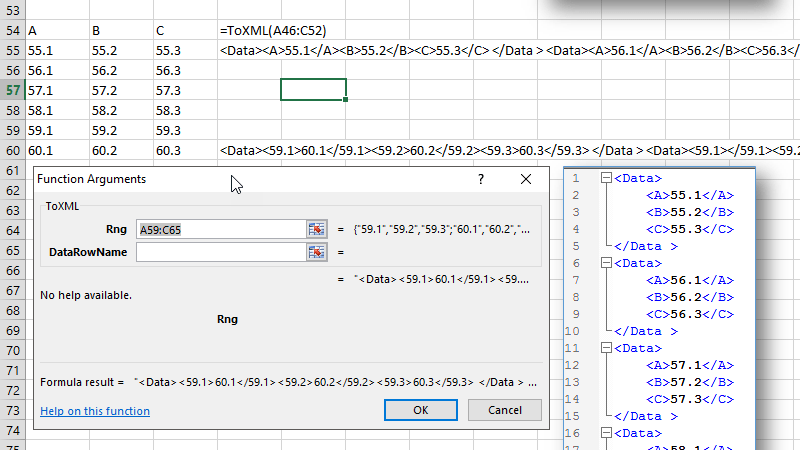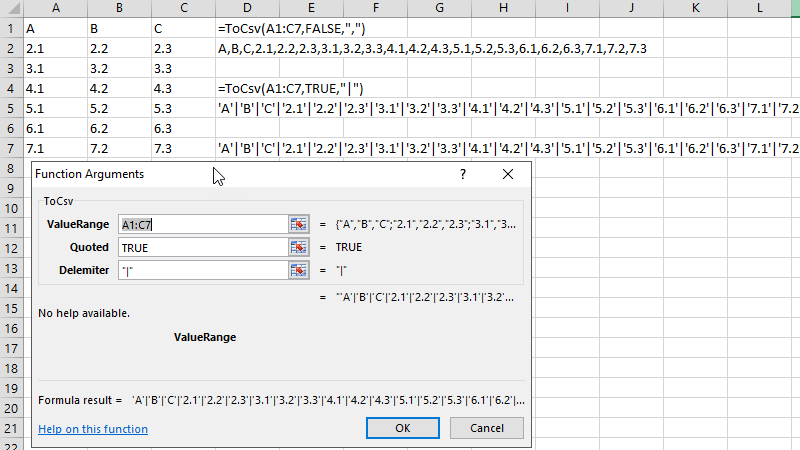# Convert Excel data to JSON CSV and XML format

By default, Excel doesn’t provide any formula to convert a range or a table into a JSON (JavaScript Object Notation), CSV (Comma Separated Value) or to an XML (Extensible Markup Language) format. This article shows how to convert a range or a table into JSON, XML, or a CSV format.

##### Convert Excel data to JSON format

Once the code below is pasted to the VBA, one can use a formula ToJSON and convert a range to a JSON format. The second argument in the formula is optional. When this argument is set to false, the name part of the JSON will NOT be surrounded by a quote as shown in the image above.

```Public Function ToJSON(table As Range, Optional quoted As Boolean = True) As String
' Make sure there are two columns in the range
If table.Columns.count < 2 Then
ToJSON = CVErr(xlErrNA)
Exit Function
End If

' Get the first row of the range as a header range

' We need to know how many columns are there
Dim colCount As Long: colCount = headerRange.Columns.count

Dim json As String: json = "["
Dim quote As String: quote = """"
If Not quoted Then quote = ""

For dataLoop = 1 To table.Rows.count
' Skip the first row as it's been used as a header
If dataLoop > 1 Then
' Start data row
Dim rowJson As String: rowJson = "{"

' Loop through each column and combine with the header
For headerLoop = 1 To colCount
rowJson = rowJson & quote & headerRange.Value2(1, headerLoop) & quote & ":"
rowJson = rowJson & """" & table.Value2(dataLoop, headerLoop) & """"
rowJson = rowJson & ","

' Strip out the last comma
rowJson = Left(rowJson, Len(rowJson) - 1)

' End data row
json = json & rowJson & "},"
End If
Next

' Strip out the last comma
json = Left(json, Len(json) - 1)

json = json & "]"

ToJSON = json
End Function
```
##### Convert Excel data to XML formatConverting to XML format is very similar to JSON format. Over the internet (client/server communication) XML format is losing ground however it is still used in web or other places.

```Public Function ToXML(rng As Range, Optional dataRowName As String = "Data") As String
' Make sure there are two columns in the range
If rng.Columns.count < 2 Then
ToXML = CVErr(xlErrNA)
Exit Function
End If

' Get the first row of the range as a header range

' We need to know how many columns are there
Dim colCount As Long: colCount = headerRange.Columns.count

Dim xml As String: xml = ""

For dataLoop = 1 To rng.Rows.count
' Skip the first row as it's been used as a header
If dataLoop > 1 Then
' Start data row
Dim rowXml As String: rowXml = xml & "<" & dataRowName & ">"

' Loop through each column and combine with the header
For headerLoop = 1 To colCount
rowXml = rowXml & CStr(rng.Value2(dataLoop, headerLoop))

' End data row
xml = rowXml & " </" & dataRowName & " > "
End If
Next

ToXML = xml
End Function```
##### Convert Excel data to CSV formatCSV format is very common format used at so many places to transport data. Eventhough CSV stands for Comman Separated Value, there are lot of other character used to separate values. So in the formula below, one can specify the delimitter character. Also if the values need to be surrounded by a single quote, the second argument can be set to true.

```Public Function ToCsv(valueRange As Range, Optional quoted As Boolean = False, Optional delemiter As String = "'") As String
Dim sb As String: sb = ""
Dim iRow, iCol As Integer

' index for loop is used to control the loop direcdtion
' with for each loop we will at mercy of application
For iRow = 1 To valueRange.Rows.count
For iCol = 1 To valueRange.Columns.count
If quoted Then
sb = sb & delemiter & "'" & valueRange.Value2(iRow, iCol) & "'"
Else
sb = sb & delemiter & valueRange.Value2(iRow, iCol)
End If

Next
Next

' Strip the last delemeter out
ToCsv = Right(sb, Len(sb) - 1)
End Function
```